# Giáo án Tiếng Anh Lớp 6 - Tiết 69, Unit 11: What do you eat ? (Lesson 2) - Năm học 2013-2014 - Ngô Thị Hương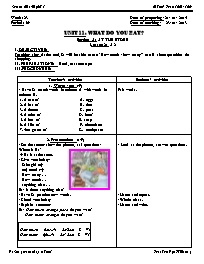I. OBJECTIVES:

Teaching aim: At the end, Ss will be able to use “How much / how many” to talk about quantities for shopping.

II. PREPARATIONS: Book, cassette tape

III. PROCEDURES:

Teacher’s activities Students’ activities

1. Warm - up: (5’)

- Have Ss match words in column A with words in column B.

1. A can of A. eggs

2. A bar of B. rice

3. A dozen C. peas

4. A tube of D. beef

5. A box of E. soap

6. A kilo of F. chocolates

7. 400 grams of G. toothpaste

2. Presentation: (15’)

- Set the scene: show the picture, ask questions:

Where is Ba?

 Ba is at the store.

- Give vocabulary:

+ Salesgirl (n):

+ (to) need (v):

+ How many

+ How much

+ anything else

Ex: Is there anything else?

- Have Ss practice new words.

- Check vocabulary

- Explain structure:

Ex: How much orange juice do you want?

How many oranges do you want?

How much + (unc.N) + do/does+ S + V?

How many+ (plu.N) + do/ does+ S + V?

3. Practice: (15’)

- Play the tape for Ss 3 times.

- Ask Ss to practice the dialogue.

- Call some pairs to practice in front of class.

- Correct the mistakes.

- Have Ss answer True/ False

1. Ba is at the market.

2. He wants some beef.

3. He wants four hundred grams of beef.

4. He wants a dozen eggs.

- Have Ss give feedback.

- Have Ss work in group, answer questions:

1. Where is Ba.

2. What does he want?

3. How much beef does he want?

4. How many eggs does he want?

- Have Ss give feedback.

4. Production: (8’)

- Have Ss draw mind map to show main idea.

- Ask Ss to work in pairs to make similar dialogue.

- Call some pairs to practice.

- Correct the mistakes.

5. Homework: (2’)

- Ask Ss to learn by heart vocabulary.

- Make 5 questions with How much / How many

Prepare lesson: Unit 11- A3, 4/ p. 117

Pair works.

- Look at the picture, answer questions.

- Listen and repeat.

- Whole class.

- Listen and write.

- Listen to the tape.

- Pair works.

- Practice.

- Listen.

- Pair works.

- Give feedback.

1. F 2. F 3. F 4. T

- Group work.

- Give feedback.

1. He is at the store.

2. He wants some beef and some eggs.

3. He wants two hundred grams of beef.

4. He wants a dozen eggs.

- Draw mind map.

- Pair work.

- Practice in front of class.

- Listen.

Take note.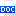2 trang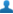lananh572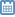03/02/2021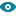1110
Bạn đang xem tài liệu "Giáo án Tiếng Anh Lớp 6 - Tiết 69, Unit 11: What do you eat ? (Lesson 2) - Năm học 2013-2014 - Ngô Thị Hương", để tải tài liệu gốc về máy bạn click vào nút DOWNLOAD ở trên
``` Week: 23 	 	 Date of preparing: 21/ 01/ 2014
Period: 69	 	 	 	 	 Date of teaching: 23/ 01/ 2013
UNIT 11: WHAT DO YOU EAT?
Section A: AT THE STORE
Lesson 2: A 2
I. OBJECTIVES:
Teaching aim: At the end, Ss will be able to use “How much / how many” to talk about quantities for shopping.
PREPARATIONS: Book, cassette tape
III. PROCEDURES:
Teacher’s activities
Students’ activities
1. Warm - up: (5’)
- Have Ss match words in column A with words in column B.
1. A can of A. eggs
2. A bar of B. rice
3. A dozen C. peas
4. A tube of D. beef
5. A box of E. soap
6. A kilo of F. chocolates
7. 400 grams of G. toothpaste
2. Presentation: (15’)
- Set the scene: show the picture, ask questions:
Where is Ba?
a Ba is at the store.
- Give vocabulary:
+ Salesgirl (n):
+ (to) need (v):
+ How many
+ How much
+ anything else
Ex: Is there anything else?
- Have Ss practice new words.
- Check vocabulary
- Explain structure:
Ex: How much orange juice do you want?
How many oranges do you want?
How much + (unc.N) + do/does+ S + V?
How many+ (plu.N) + do/ does+ S + V?
3. Practice: (15’)
- Play the tape for Ss 3 times.
- Ask Ss to practice the dialogue.
- Call some pairs to practice in front of class.
- Correct the mistakes.
- Have Ss answer True/ False
1. Ba is at the market.
2. He wants some beef.
3. He wants four hundred grams of beef.
4. He wants a dozen eggs.
- Have Ss give feedback.
- Have Ss work in group, answer questions:
1. Where is Ba.
2. What does he want?
3. How much beef does he want?
4. How many eggs does he want?
- Have Ss give feedback.
4. Production: (8’)
- Have Ss draw mind map to show main idea.
Ba wants
- Ask Ss to work in pairs to make similar dialogue.
- Call some pairs to practice.
- Correct the mistakes.
5. Homework: (2’)
- Ask Ss to learn by heart vocabulary.
- Make 5 questions with How much / How many
Prepare lesson: Unit 11- A3, 4/ p. 117
Pair works.
- Look at the picture, answer questions.
- Listen and repeat.
- Whole class.
- Listen and write.
- Listen to the tape.
- Pair works.
- Practice.
- Listen.
- Pair works.
- Give feedback.
1. F 2. F 3. F 4. T
- Group work.
- Give feedback.
1. He is at the store.
2. He wants some beef and some eggs.
3. He wants two hundred grams of beef.
4. He wants a dozen eggs.
- Draw mind map.
- Pair work.
- Practice in front of class.
- Listen.
Take note.
•Tuan 23 E6 tiet 69Unit 11 A2.doc